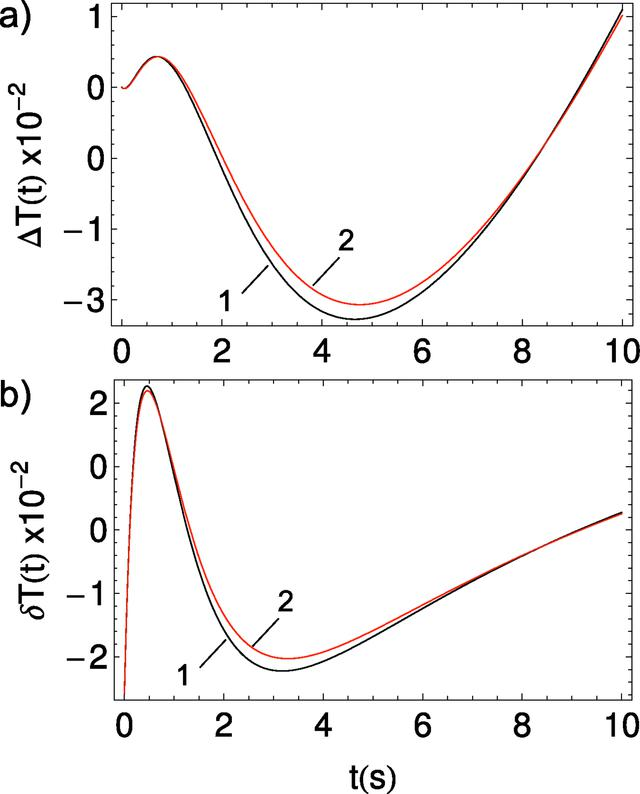disable zoom     view article Figure 8 Differences of T(t) estimates. (a) Absolute. (b) Relative. In (a) the black solid curve is the absolute difference of model 2 minus model 1 applied for the exact calculation of T(t); the red solid line is the absolute difference of model 2 minus model 1 applied for the T(t) approximation. Plots in (b) correspond to plots presented in (a) divided by T(t) calculated for model 2 for the exact and approximated cases, respectively.JOURNAL OFSYNCHROTRONRADIATION
ISSN: 1600-5775
Volume 25| Part 3| May 2018| Pages 833-847